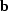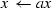Next: Operator Up: A deconvolution example Previous: A deconvolution example

## Vectors

The observed data, the known blurring filter, and the unknown deblurred imageare vectors. Each of them is accompanied by their own vector space. Vector and vector space are Java interfaces that define the basic vector operations, such as listed in Table 2 and Table 3.

 add x.add(y)scale x.scale(a)dot a = x.dot(y)getSpace x.getSpace() returns vector space

 hasMember s.hasMember(x) true if vector x is member newMember x = s.newMember() creates a new member x

In the deconvolution example, the various vectors are represented by Rsf (Regularly Sampled Function) objects. The Rsf class is implemented as a multi-dimensional, in-core array. The RsfSpace class is an array of physical Axis descriptions. Rsf and RsfSpace are, of course, implementations of the general vector and space interface. and consequently implement the basic vector methods of Table 2 and Table 3. The Rsf and RsfSpace classes provide additional features, but for the moment it suffices to know that an Rsf contains a write(filename) method that stores a representation of itself and its vector space in a disk file. A class RsfFactory can read such a disk file and create the corresponding Rsf.Next: Operator Up: A deconvolution example Previous: A deconvolution example
Stanford Exploration Project
3/8/1999